Publicité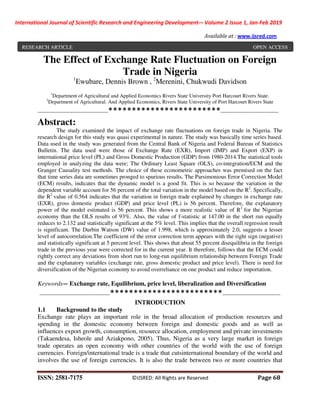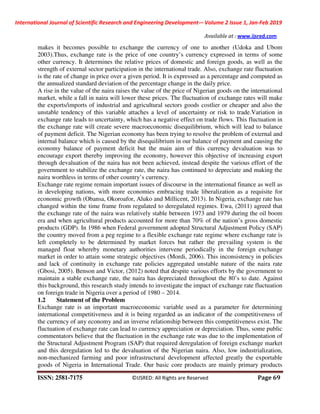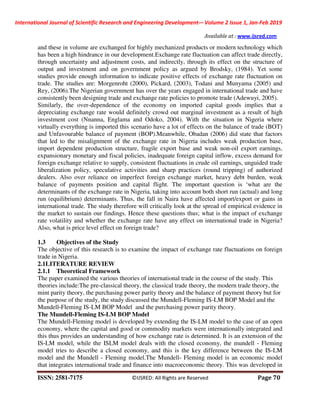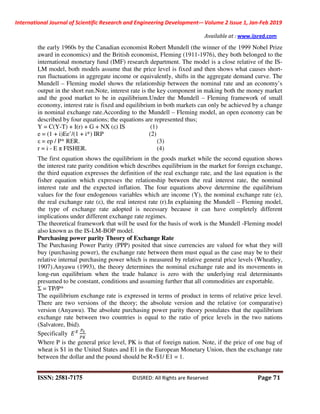Publicité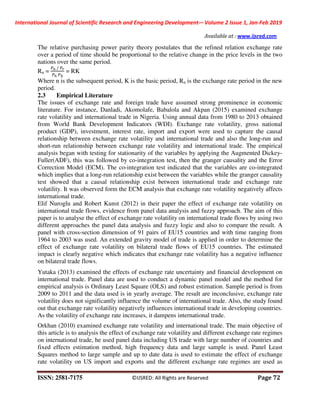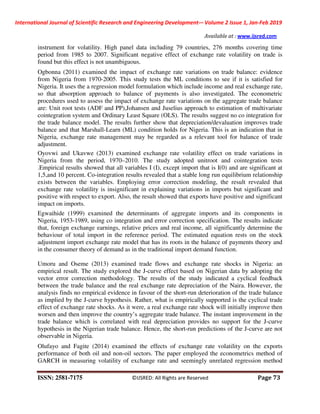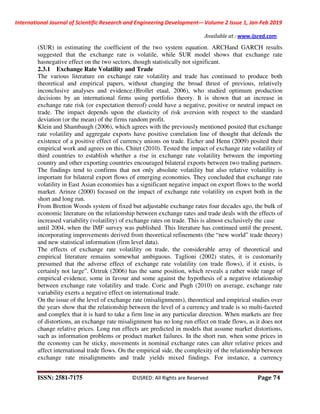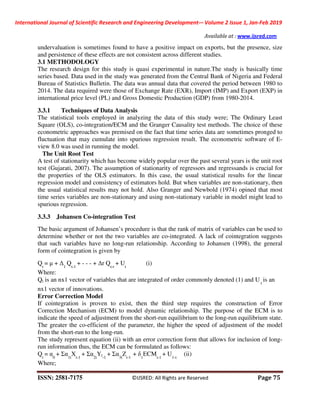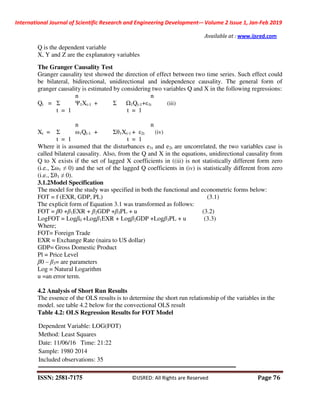Publicité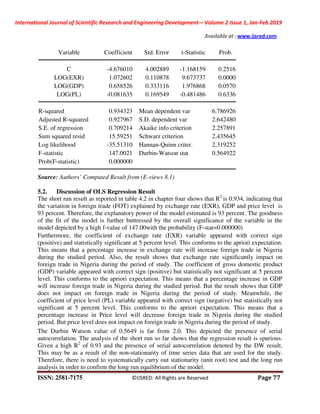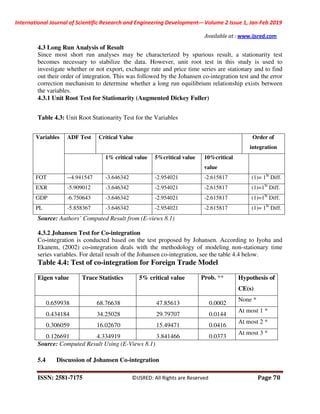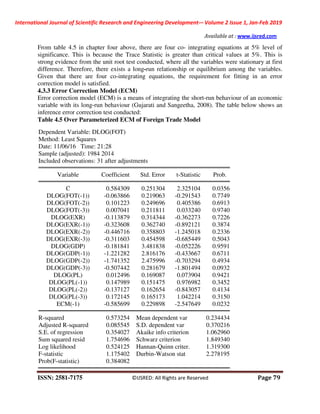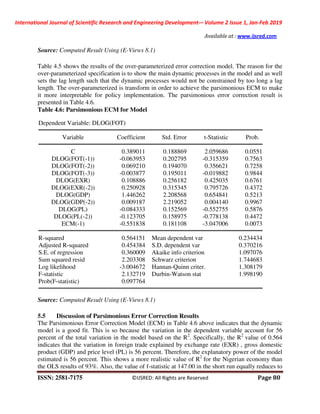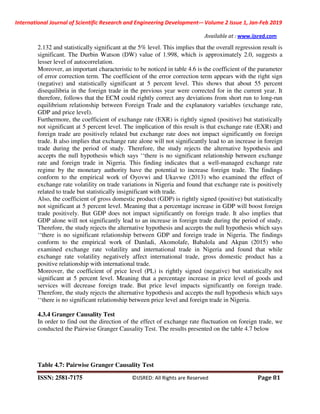Publicité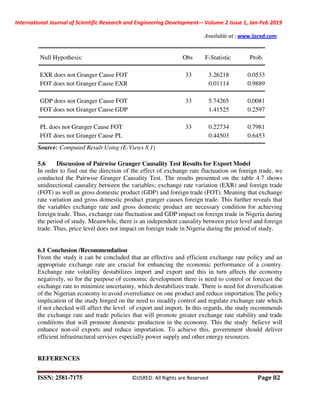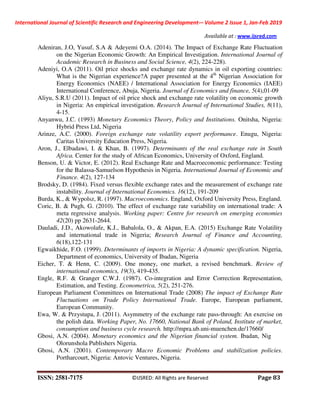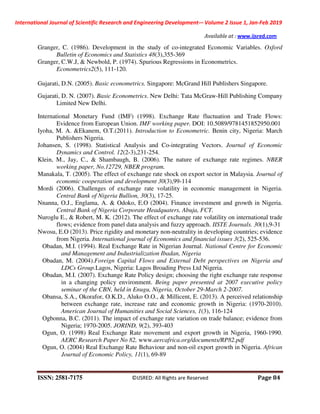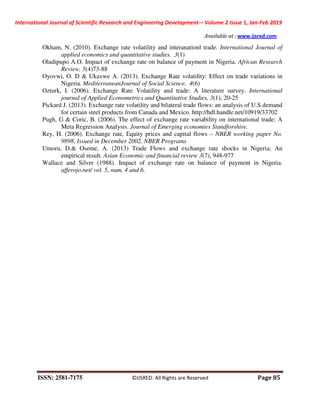Prochain SlideShare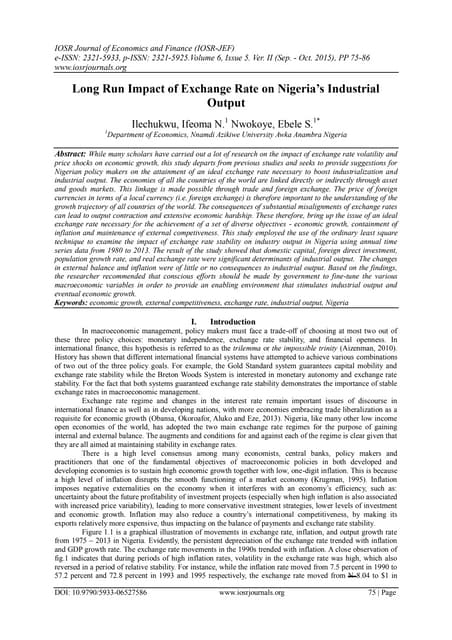Long Run Impact of Exchange Rate on Nigeria’s Industrial Output
Chargement dans ... 3
1 sur 18
Publicité

### IJSRED-V2I1P11

4. International Journal of Scientific Research and Engineering Development-– Volume 2 Issue 1, Jan-Feb 2019 Available at : www.ijsred.com ISSN: 2581-7175 ©IJSRED: All Rights are Reserved Page 71 the early 1960s by the Canadian economist Robert Mundell (the winner of the 1999 Nobel Prize award in economics) and the British economist, Fleming (1911-1976), they both belonged to the international monetary fund (IMF) research department. The model is a close relative of the IS- LM model, both models assume that the price level is fixed and then shows what causes short- run fluctuations in aggregate income or equivalently, shifts in the aggregate demand curve. The Mundell – Fleming model shows the relationship between the nominal rate and an economy’s output in the short run.Note, interest rate is the key component in making both the money market and the good market to be in equilibrium.Under the Mundell – Fleming framework of small economy, interest rate is fixed and equilibrium in both markets can only be achieved by a change in nominal exchange rate.According to the Mundell – Fleming model, an open economy can be described by four equations; the equations are represented thus; Y = C(Y-T) + I(r) + G + NX (ε) IS (1) e = (1 + i)Ee’/(1 + i*) IRP (2) ε = ep / P* RER. (3) r = i - E π FISHER. (4) The first equation shows the equilibrium in the goods market while the second equation shows the interest rate parity condition which describes equilibrium in the market for foreign exchange, the third equation expresses the definition of the real exchange rate, and the last equation is the fisher equation which expresses the relationship between the real interest rate, the nominal interest rate and the expected inflation. The four equations above determine the equilibrium values for the four endogenous variables which are income (Y), the nominal exchange rate (e), the real exchange rate (ε), the real interest rate (r).In explaining the Mundell – Fleming model, the type of exchange rate adopted is necessary because it can have completely different implications under different exchange rate regimes. The theoretical framework that will be used for the basis of work is the Mundell -Fleming model also known as the IS-LM-BOP model. Purchasing power parity Theory of Exchange Rate The Purchasing Power Parity (PPP) posited that since currencies are valued for what they will buy (purchasing power), the exchange rate between them must equal as the case may be to their relative internal purchasing power which is measured by relative general price levels (Wheatley, 1907).Anyawu (1993), the theory determines the nominal exchange rate and its movements in long-run equilibrium when the trade balance is zero with the underlying real determinants presumed to be constant, conditions and assuming further that all commodities are exportable. Σ = TP/P* The equilibrium exchange rate is expressed in terms of product in terms of relative price level. There are two versions of the theory; the absolute version and the relative (or comparative) version (Anyawu). The absolute purchasing power parity theory postulates that the equilibrium exchange rate between two countries is equal to the ratio of price levels in the two nations (Salvatore, Ibid). Specifically Where P is the general price level, PK is that of foreign nation. Note, if the price of one bag of wheat is $1 in the United States and E1 in the European Monetary Union, then the exchange rate between the dollar and the pound should be R=$1/ E1 = 1.